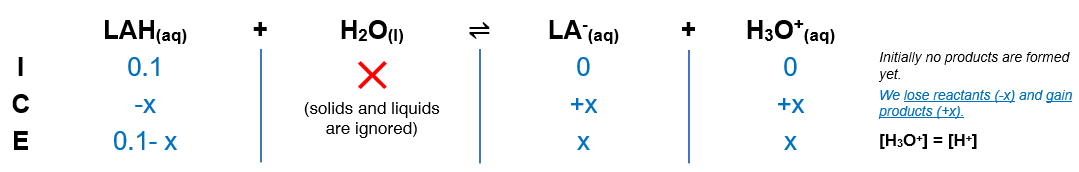# Problem: Lactic acid is a common by-product of cellular respiration and is often said to cause the “burn” associated with strenuous activity. A 25.0-mL sample of 0.100 M lactic acid (HC3H5O3, pKa = 3.86) is titrated with 0.100 M NaOH solution. Calculate the pH after the addition of 0.0 mL

🤓 Based on our data, we think this question is relevant for Professor Simon's class at ULL.

###### FREE Expert Solution

(1) Construct an ICE chart(2) Calculate x

If [HA]/Ka is << 500; -x is negligible

0.1/1.38x10-4 = 724 < 500; Hence -x is negligible###### Problem Details

Lactic acid is a common by-product of cellular respiration and is often said to cause the “burn” associated with strenuous activity. A 25.0-mL sample of 0.100 M lactic acid (HC3H5O3, pKa = 3.86) is titrated with 0.100 M NaOH solution. Calculate the pH after the addition of 0.0 mL